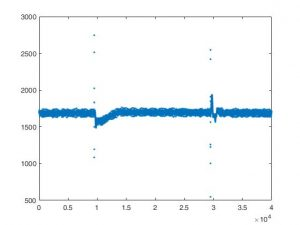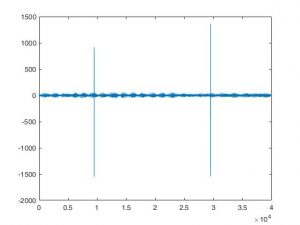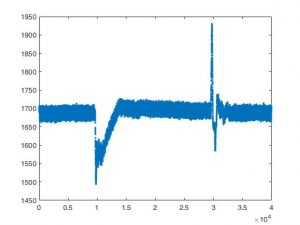# 关于Matlab 读取数据文件

``A = readmatrix(filename)``
``A = readmatrix(filename,opts)``
``A = readmatrix(___,Name,Value)``
opts参数对于这个命令比较重要，在处理excel文件时比较有效。在使用该命令的时候可以采用预览模式
opts = detectImportOptions(‘airlinesmall_subset.xlsx’);
preview(‘airlinesmall_subset.xlsx’,opts)

```opts.Sheet = '2007';
opts.SelectedVariableNames = [1:5];
opts.DataRange = '2:11';

# 新版本的ABAQUS2MATLAB发布了

Abaqus2Matlab作为源代码免费发布，目的是促进研究。

Abaqus2Matlab v2.0 for 32-bit Windows has just been released!
The software that connects Abaqus to Matlab and vice versa can now be used in Windows 32-bit platforms, aiming to accelerate the pace of research and engineering. You can now download the 32-bit version including but not limited to the 64-bit version, documentation, examples and getting started guide, all for free, from the official website of the software: http://www.abaqus2matlab.com/
Thanks to all the hard-working Abaqus2Matlab community who have contributed to various improvements of the software by sharing their experience, identifying bugs, and proving that Abaqus2Matlab can be used for a very broad scope of applications for engineering research!

Ask, discuss or suggest your problems and/or experiences with Abaqus2Matlab here: http://abaqus2matlab.wixsite.com/abaqus2matlab/forum/abaqus2matlab-users-forum
View the original announcement here: http://abaqus2matlab.wixsite.com/abaqus2matlab/forum/news-about-abaqus2matlab/abaqus2matlab-v2-0-for-32-bit-windows-has-just-been-released

A novel software is presented to connect Abaqus, a sophisticated finite element package, with Matlab, the most comprehensive program for mathematical analysis.
This interface between these well-known codes not only benefits from the image processing and the integrated graph-plotting features of Matlab, but opens up new opportunities in results post-processing, statistical analysis, and mathematical optimization, among many other possibilities.
Abaqus2Matlab is freely distributed as source code with the aim of facilitating research.

# 再论MATLAB的力学传感器测量信号处理，消除脉冲噪音```function new_data=flat_data(ori_data)
% the difference of the data point is fixed and is equal to 1
% then we will measure the difference of pressure between two data points
n_length=length(ori_data);
diff_y=abs(diff(ori_data));% absolute value
new_data=ori_data;% data initialization
span_end=1;
flag_start=1;
for i_data=1:n_length-1
if diff_y(i_data)>100&&flag_start==1
snap_start=i_data;
span_end=n_length;
flag_start=0;
else
if span_end>1&&diff_y(i_data)<100
snap_end=i_data;
length_span=snap_end-snap_start;
span_y=ori_data(snap_end)-ori_data(snap_start);
if abs(span_y)>0
span_dif=span_y/length_span;
new_data_rep=ori_data(snap_start):span_dif:ori_data(snap_end);% linear fitting
new_data(snap_start:snap_end)=new_data_rep;
else
new_data_rep=ones(length_span+1,1)*ori_data(snap_start);
new_data(snap_start:snap_end)=new_data_rep;
end
flag_start=1;
span_end=1;
end
end
end

```

# Matlab 一维中值滤波（信号处理）

matlab 中值滤波函数 medfilt1函数用于一维信号的处理。例如在动态压力测量时由于电路上的干扰会出现测量值发生突变，为了消除这种突变可以采用一维中值滤波的方法。

• ``y = medfilt1(x), 对于输入的向量采用3阶的一维滤波``
• ``y = medfilt1(x,n)，对于输入的向量采用n阶的一维滤波``
`效果如下图（MATLAB  help  medfilt1 参考页）。`